# Catenary

(diff) ← Older revision | Latest revision (diff) | Newer revision → (diff)
Jump to: navigation, search

The plane transcendental curve describing the form of a homogeneous flexible string of fixed length and with fixed ends attained under the action of gravity (see Fig.).Figure: c020790a

In Cartesian coordinates its equation is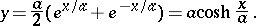The length of an arc beginning at the point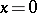is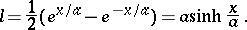The radius of curvature is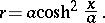The area bounded by an arc of the catenary, two of its ordinates and the-axis is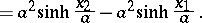If an arc of a catenary is rotated around the-axis, it forms a catenoid.

How to Cite This Entry:
Catenary. Encyclopedia of Mathematics. URL: http://encyclopediaofmath.org/index.php?title=Catenary&oldid=16508
This article was adapted from an original article by D.D. Sokolov (originator), which appeared in Encyclopedia of Mathematics - ISBN 1402006098. See original article